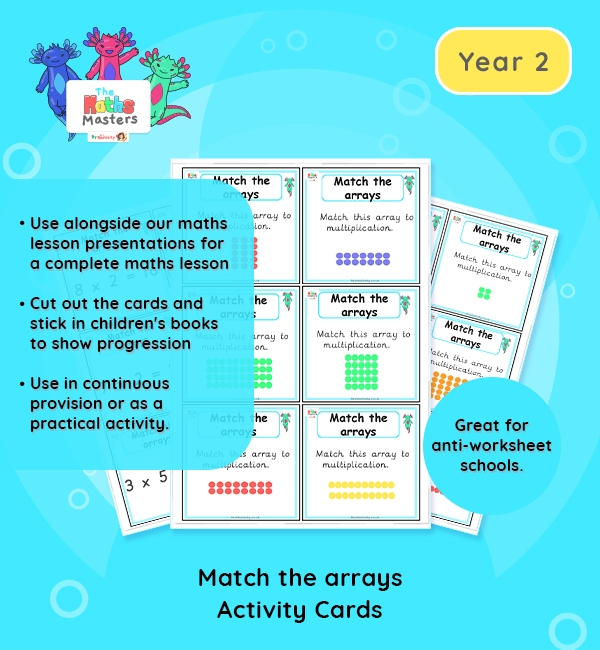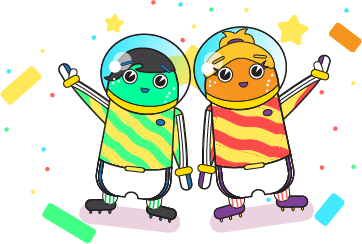# Year 2 | Using Arrays Activity Cards## Year 2 multiplication activity cards

Aligned with the maths mastery approach, these Year 2 | Using Arrays Activity Cards are designed to be used alongside our Year 2 | Using Arrays Lesson Presentation for a complete maths lesson. The maths activity cards requires children match the array to the written multiplication card. The cards should be clear enough for children to do independently, once the learning has been modelled in the above presentation. These cards are also brilliant for sticking in children’s books alongside photographs, to demonstrate learning and progression. Great for anti-worksheet schools!

Topic: Multiplication and division

Small Steps: Use arrays.

NC Links: Recall and use multiplication and division facts for the 2, 5, and 10 times tables. *Calculate mathematical statements for multiplication and division within the multiplication tables and write them using the multiplication, division and equals signs. *Solve problems involving multiplication and division using materials, arrays, repeated addition, mental methods and multiplication and division facts including problems in context. *Count in steps of 2, 3, 5, and 10. *Show that the multiplication of two numbers can be done in any order.

TAF Statements: Working At  – Recall and use multiplication and division facts for 2, 5, and 10 and use them to solve simple problems.

Greater Depth – Use reasoning about number and relationships to solve more complex problems and explain their thinking.

Ready-to-progress criteria:  2MD-1 Recognise repeated addition contexts, representing them with multiplication equations and calculating the product within the 2, 5, and 10 multiplication tables.

Year 1 conceptual prerequisites: Count in multiples of 2, 5 and 10

Future applications: Use multiplication to represent repeated addition contexts for other group sizes.

## Recently Viewed